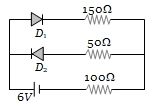NEET Physics Semiconductor Electronics Questions Solved

The circuit shown in following figure contains two diode ${\mathrm{D}}_{1}$ and ${\mathrm{D}}_{2}$ each with a forward resistance of 50 ohms and with infinite backward resistance. If the battery voltage is 6 V, the current through the 100 ohm resistance (in amperes) is(a) Zero                     (b) 0.02
(c) 0.03                     (d) 0.036

Explanation is a part of a Paid Course. To view Explanation Please buy the course.

Difficulty Level: